# Thick BeamThe purpose of this test is to check membrane elements. You will use 2D meshes.Reference:

M. ZIENKIEWICKZ, The Finite Element Method, 4th edition, Vol. 1, p.386.

##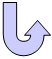Specifications

### Geometry Specifications

 Length: L = 10 m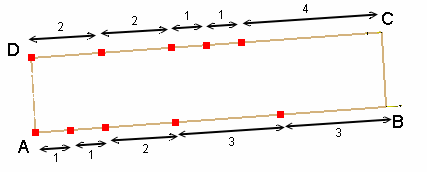Width = W = 2 m Thickness: th = 0.001 m

### Analysis Specifications

 Young Modulus (material): E = 1500 MPa Poisson's Ratio (material): ν = 0.25 Mesh Specifications: Mesh size: 4000 mm Constrained points (using the points created in the CATPart) Membrane elements Restraints (User-defined): On edge AD, Tx = 0 At point A, Tx = Ty = 0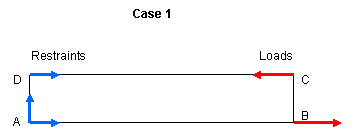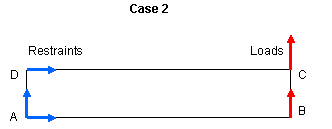Loads (Distributed force): Case 1: FX = 1000 N at point B FX = -1000 N at point C Case 2: Fy = 150 N at points B and C

##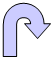Results

• The analytical solution is the vertical displacement at point B. The corresponding analytical solutions are:
Case 1: Ty = 100.00 mm
Case 2: Ty = 102.60 mm

• The table below presents the analysis results.
The results correspond to the vertical displacement at point B.
The normalized results (computed results divided by analytical solution) are listed.

 Analytical solution [mm] Values Linear triangle (TR3) Parabolic triangle (TR6) Linear quadrangle (QD4) Parabolic quadrangle (QD8)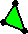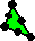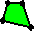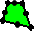Computed results  [mm] Normalized results Computed results  [mm] Normalized results Computed results  [mm] Normalized results Computed results  [mm] Normalized results Case 1 100 25.49 0.255 100 1.000 96.18 0.962 100 1.000 Case 2 102.6 29.78 0.290 101.54 0.989 98.05 0.956 102.8 1.002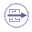To Perform the Test:

The thick_beam_qd8.CATAnalysis document presents a complete analysis of this case, computed with a mesh formed of parabolic quadrangle elements (QD8).

To compute the case with other types of elements, proceed as follow:

1. Open the CATAnalysis document.

2. In the Advanced Meshing Tools workbench, change the mesh specifications as indicated above.

3. Compute the case in the Generative Structural Analysis workbench.